# The MI Procedure

### Specifying Sets of Observations for Imputation in Pattern-Mixture Models

By default, all available observations are used to derive the imputation model. By using the MODEL option in the MNAR statement, you can specify the set of observations that are used to derive the model. You specify a classification variable (obs-variable) by using the option MODELOBS= (obs-variable= level1’ <’level2’ …>). The MI procedure uses the group of observations for which obs-variable equals one of the specified classification levels.

When you use the MNAR statement together with a MONOTONE statement, you can also use the MODELOBS=CCMV and MODELOBS=NCMV options to specify the set of observations for deriving the imputation model. For a monotone missing pattern data set that contains the variables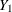,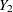, …,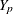(in that order), there are at most p groups of observations such that the same number of variables is observed for observations in each group. The complete-case missing values (CCMV) method (Little 1993; Molenberghs and Kenward 2007, p. 35) uses the group of observations for which all variables are observed (complete cases) to derive the imputation model. The neighboring-case missing values (NCMV) method (Molenberghs and Kenward, 2007, pp. 35–36) uses only the neighboring group of observations (that is, for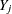, the group of observations withobserved and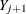missing).

In PROC MI, the option MODELOBS=CCMV(K=k) uses the k groups of observations together with as many observed variables as possible to derive the imputation model. For instance, specifying K=1 (which is the default) uses observations from the group that has all variables observed (complete cases). Specifying K=2 uses observations from the two groups that have the most variables observed (the group of observations that has all variables observed and the group of observations that hasobserved butmissing).

For an imputed variable, the option MODELOBS=NCMV(K=k) uses the k closest groups of observations that have observedbut have as few observed variables as possible to derive the imputation model. For instance, specifying K=1 (which is the default) uses the group of observations that hasobserved butmissing (neighboring cases). Specifying K=2 uses observations from the two closest groups that haveobserved (the group of observations that hasobserved butmissing, and the group of observations that hasobserved and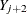missing).

When you use the MNAR statement together with an FCS statement, the MODEL option applies only after the preliminary filled-in phase in each of the imputations.

For an illustration of the MODEL option, see Example 63.15.